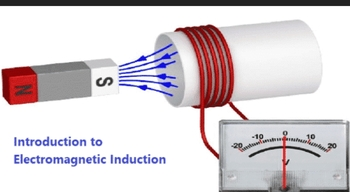Introduction to Electromagnetic InductionWhat is Electromagnetic Induction?

Electromagnetic Induction was discovered by Michael Faraday in 1831 and James Clerk Maxwell mathematically described it as Faraday’s law of induction.

Electromagnetic Induction is a current produced because of voltage production (electromotive force) due to a changing magnetic field.

This either happens when a conductor is placed in a moving magnetic field (when using AC power source) or when a conductor is constantly moving in a stationary magnetic field.

Michael Faraday arranged a conducting wire as per the setup given below, attached to a device to measure the voltage across the circuit. When a bar magnet was moved through the coiling, the voltage detector measures the voltage in the circuit.Through his experiment, he discovered that there are certain factors that influence this voltage production. They are:

1. Number of Coils: The induced voltage is directly proportional to the number of turns/coils of the wire. Greater the number of turns, greater is voltage produced
2. Changing Magnetic Field: Changing magnetic field affects the induced voltage. This can be done by either moving the magnetic field around the conductor or moving the conductor in the magnetic field.